Test: Magnetically Coupled Circuits- 2

# Test: Magnetically Coupled Circuits- 2

Test Description

## 10 Questions MCQ Test Topicwise Question Bank for Electrical Engineering | Test: Magnetically Coupled Circuits- 2

Test: Magnetically Coupled Circuits- 2 for Electrical Engineering (EE) 2022 is part of Topicwise Question Bank for Electrical Engineering preparation. The Test: Magnetically Coupled Circuits- 2 questions and answers have been prepared according to the Electrical Engineering (EE) exam syllabus.The Test: Magnetically Coupled Circuits- 2 MCQs are made for Electrical Engineering (EE) 2022 Exam. Find important definitions, questions, notes, meanings, examples, exercises, MCQs and online tests for Test: Magnetically Coupled Circuits- 2 below.
Solutions of Test: Magnetically Coupled Circuits- 2 questions in English are available as part of our Topicwise Question Bank for Electrical Engineering for Electrical Engineering (EE) & Test: Magnetically Coupled Circuits- 2 solutions in Hindi for Topicwise Question Bank for Electrical Engineering course. Download more important topics, notes, lectures and mock test series for Electrical Engineering (EE) Exam by signing up for free. Attempt Test: Magnetically Coupled Circuits- 2 | 10 questions in 30 minutes | Mock test for Electrical Engineering (EE) preparation | Free important questions MCQ to study Topicwise Question Bank for Electrical Engineering for Electrical Engineering (EE) Exam | Download free PDF with solutions
 1 Crore+ students have signed up on EduRev. Have you?
Test: Magnetically Coupled Circuits- 2 - Question 1

### The overall inductance of two coils connected in series, with mutual inductance opposing self-inductance is L1; with mutual inductance aiding self-inductance the overall inductance is L2. The mutual inductance M is given by

Detailed Solution for Test: Magnetically Coupled Circuits- 2 - Question 1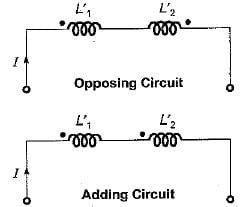Let the self inductances of two coils be  L'and L'2 respectively.
Then, L1 = L'1 + L'2 - 2M (For opposing)
and L2 = L'1 +  L'2  + 2M (For adding)
So, L2 - L1 = 4M
or,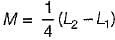Test: Magnetically Coupled Circuits- 2 - Question 2

### In case all the flux from the current in coil-1 links with coil-2, the coefficient of coupling will be

Test: Magnetically Coupled Circuits- 2 - Question 3

### In series circuit shown below, for series resonant frequency of 1 rad/s, the value of coupling coefficient K will be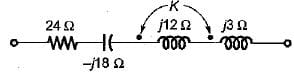Detailed Solution for Test: Magnetically Coupled Circuits- 2 - Question 3

Using coupling coefficient formula, wo have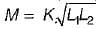Now, for resonance,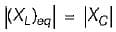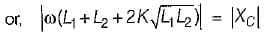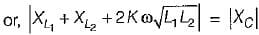or,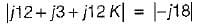or, 15 + 12 k = 18
or, K = 3/12 = 0.25

Test: Magnetically Coupled Circuits- 2 - Question 4

The equivalent inductance for the following inductive circuit is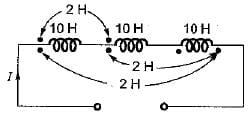Detailed Solution for Test: Magnetically Coupled Circuits- 2 - Question 4

Leq = (10 + 2 - 2) + (10 + 2 - 2)+ (10 + 2 - 2) = 30 H

Test: Magnetically Coupled Circuits- 2 - Question 5

The resonant frequency of the given series circuit is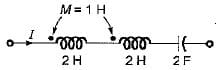Detailed Solution for Test: Magnetically Coupled Circuits- 2 - Question 5

Leq = L + L + 2 M
= 2 + 2 + 2 x 1 = 6 H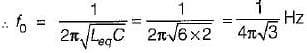Test: Magnetically Coupled Circuits- 2 - Question 6

A 3 H inductor has 1000 turns. What should be the number of turns to increase the inductance to 5 H?

Detailed Solution for Test: Magnetically Coupled Circuits- 2 - Question 6

We know that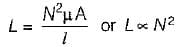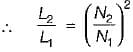or,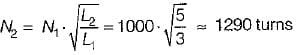Test: Magnetically Coupled Circuits- 2 - Question 7

What is the equivalent inductance between the terminals A and B for the circuit shown below? (Given, M = 1H)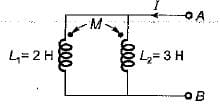Detailed Solution for Test: Magnetically Coupled Circuits- 2 - Question 7

Due to same polarity, the effect of mutual inductance will he positive.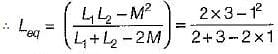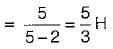Test: Magnetically Coupled Circuits- 2 - Question 8

Assertion (A): To determine the relative polarity of the induced voltage in the coupled coil, the coils are marked with dots.
Reason (R): One each coil, a dot is placed at the terminals which are instantaneously of the same polarity on the basis of mutual inductance alone.

Test: Magnetically Coupled Circuits- 2 - Question 9

Assertion (A): When a current changes in a circuit, the magnetic fiux linking the same circuit changes and an emf is induced in the circuit.
Reason (R): Mutual inductances is the bilateral property of the linked circuit.

Detailed Solution for Test: Magnetically Coupled Circuits- 2 - Question 9

The reason for assertion is that, emf is induced due to Faraday’s law of electromagnetic induction. Reason (R) and assertion (A) are individually true.

Test: Magnetically Coupled Circuits- 2 - Question 10

Match List- I (Connection) with List-ll (Equivalent inductance) and select the correct answer using the codes given below the lists: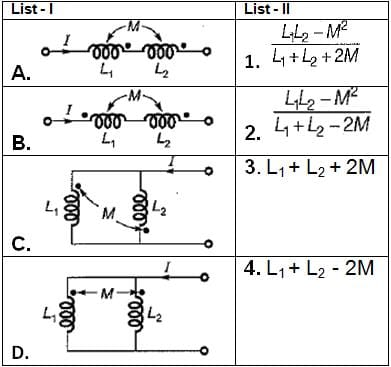Codes: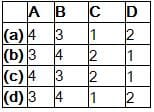## Topicwise Question Bank for Electrical Engineering

147 tests
 Use Code STAYHOME200 and get INR 200 additional OFF Use Coupon Code
Information about Test: Magnetically Coupled Circuits- 2 Page
In this test you can find the Exam questions for Test: Magnetically Coupled Circuits- 2 solved & explained in the simplest way possible. Besides giving Questions and answers for Test: Magnetically Coupled Circuits- 2, EduRev gives you an ample number of Online tests for practice

147 tests